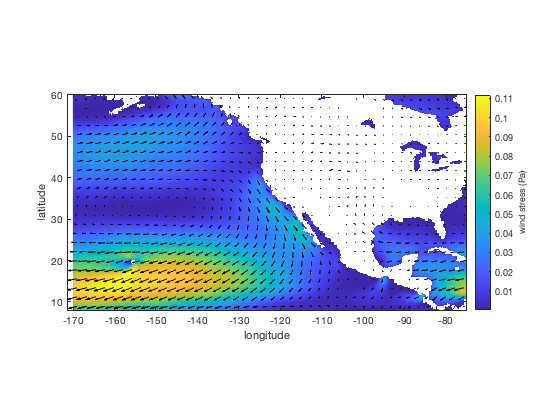# windstress documentation

The windstress function estimates wind stress on the ocean from wind speed.

Back to Climate Data Tools Contents

## Syntax

```Tau = windstress(u10)
[Taux,Tauy] = windstress(u10,v10)
[...] = windstress(...,'Cd',Cd)
[...] = windstress(...,'ci',seaIce)
[...] = windstress(...,'rho',airDensity)```

## Description

Tau = windstress(u10) estimates the wind stress (in pascals) imparted on the ocean by the wind speed (in meters per second) 10 m above the surface. Output Tau is the same size as input u10.

[Taux,Tauy] = windstress(u10,v10) simultaneously computes zonal and meridional components of wind stress.

[...] = windstress(...,'Cd',Cd) specifies a coefficient of friction Cd. Default Cd is 1.25e-3, which is a global average (Kara et al., 2007) but in reality Cd can vary quite a bit in space and time. Cd can be a scalar or a vector, 2D matrix, or 3D matrix the same size as u10 (and v10 if v10 is included).

[...] = windstress(...,'ci',seaIce) specifies sea ice concentration for estimation of Cd as given by Lüpkes and Birnbaum, 2005. Input seaIce is a fraction of sea ice cover and must be in the range 0 to 1 inclusive. seaIce can be a scalar or a vector, 2D matrix, or 3D matrix the same size as u10 (and v10 if v10 is included).

[...] = windstress(...,'rho',airDensity) specifies air density, which can be a scalar or a vector, 2D matrix, or 3D matrix the same size as u10 (and v10 if v10 is included). Default value of airDensity is 1.225 kg/m^3.

## Example 1a: Estimate wind stress from reanalysis data

Load the sample pacific_wind.mat, then plot the wind stress on the ocean resulting from the wind. Below I'm using the sst dataset to mask out land values, but you could just as easily use island if there weren't already this easy way to distinguish land from ocean in the dataset.

We'll use imagescn to plot wind stress and quiversc to plot the wind vectors:

```load pacific_wind

% Calculate magnitude of wind stress from wind speed
Tau = windstress(hypot(u10,v10));

% Mask-out land values by setting them to NaN:
Tau(isnan(sst)) = NaN;

% Plot with imagesn, imagesc, or pcolor:
figure
imagescn(lon,lat,Tau)
axis xy image
cb = colorbar;
ylabel(cb,' wind stress (Pa) ')
xlabel('longitude')
ylabel('latitude')

hold on
quiversc(lon,lat,u10,v10,'k')
```## Example 1b: Be careful with components

There's a funny thing about wind stress, which is its magnitude is proportional to the square of wind speed. If you calculate each component (zonal and meridional) of wind stress individually from each component of wind speed (u10 and v10), the resulting wind stress vectors can have an incorrect magnitude and direction!

Consider a velocity vector whose components are u10 = 2 m/s and v10 = 1 m/s. From this we know the velocity vector has an angle atand(1/2) = 26.6 degrees. Wind stress is proportional to the square of wind speed, but if we square each component of the wind speed to obtain a wind stress vector, the resulting wind stress vector will not even point in the right direction! Squaring each component of wind speed in this example would give a wind stress vector with an angle atand(1/4) = 14.0 degrees. And its magnitude would be incorrect too.

Compare the correctly-computed wind stress vectors to the incorrectly-computed wind stress vectors by placing correct vectors (blue) and incorrect vectors (red) on top of the black wind velocity vectors from Example 1a:

```% Calculate correct wind stress components:
[Taux,Tauy] = windstress(u10,v10);

% Plot correct blue wind stress vectors atop the black wind velocity vectors:
quiversc(lon,lat,Taux,Tauy,'b')
% Incorrectly calculate wind stress as components:
Taux_wrong = windstress(u10);
Tauy_wrong = windstress(v10);

% Plot incorrect red wind stress vectors:
quiversc(lon,lat,Taux_wrong,Tauy_wrong,'r')

% Zoom in:
axis([-145 -110 15 40])
```Above, the correct (blue) wind stress vectors align with the black wind velocity vectors, whereas computing wind stress components individually from wind speed components produces red wind stress vectors of incorrect magnitude and direction.

## Example 2: (Theoretical) effect of sea ice

Explore the relationship between wind speed, sea ice concentration, and wind stress. Start by defining values of wind speed from 0 to 25 m/s, and sea ice concentration from 0 to 1, then calculate wind stress and plot it as a function of wind speed and sea ice concentration:

```% Define some values of wind speed and sea ice concentration:
[U,ci] = meshgrid(0:0.1:25,0:0.01:1);

% Calculate wind stress:
Tau = windstress(U,'ci',ci);

% Plot wind stress as a fn of wind speed and sea ice concentration:
figure
imagescn(U,ci,Tau)
xlabel('10 m wind speed (m/s)')
ylabel('sea ice concentration')
cb = colorbar;
ylabel(cb,' wind stress (Pa) ')
```Note how at first wind stress increases with sea ice concentration because the jagged edges of the sea ice give the wind something to grab onto, but when the sea ice concentration exceeds 63%, the clogged ice field begins to prevent the wind from transferring energy to the water.

## References

Kara, A. Birol, et al. "Wind stress drag coefficient over the global ocean." Journal of Climate 20.23 (2007): 5856-5864. doi:10.1175/2007JCLI1825.1.

Lüpkes, Christof, and Gerit Birnbaum. "Surface drag in the Arctic marginal sea-ice zone: a comparison of different parameterisation concepts." Boundary-layer meteorology 117.2 (2005): 179-211. doi:10.1007/s10546-005-1445-8.

## Author Info

The windstress function and supporting documentation were written by Chad A. Greene of the University of Texas at Austin, Institute for Geophysics (UTIG), February 2017.